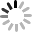产品速递 | 青铜剑推出适配1000V以下NPC1型三电平系统的紧凑型驱动器

2021-03-05 13:42

1000V以下NPC1型三电平中小功率DC/AC变流系统具有高集成度、高功率密度、低成本的特点，青铜剑技术针对该系统设计推出配套门极驱动器2QD0108T06-SE2。

2QD0108T06-SE2是一款紧凑型、即插即用的双通道驱动器，采取竖插装形式，相比同类产品尺寸减小50%以上，可以显著减小对主PCB的面积的占用，提高主PCB的面积的利用率。该产品可适配市面上大部分采用Easy封装的650V NPC1型三电平模块，以及采用单管形式的三电平拓扑，亦适用于普通两电平的拓扑，具有很好的通用性。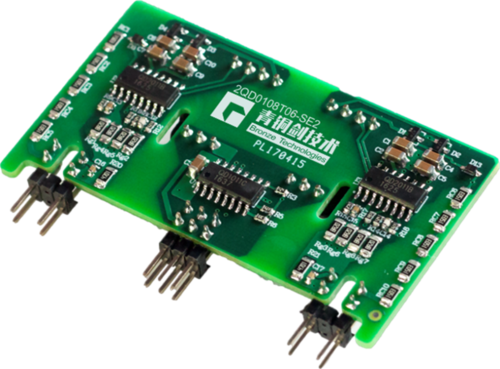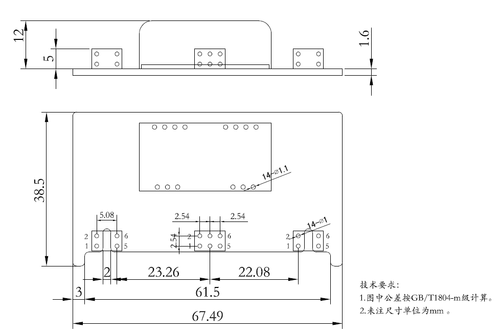IGBT短路保护功能，保护时间＜7.0us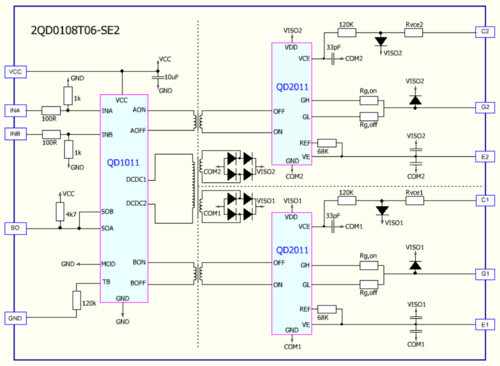IGBT：F3L150R07W2E3_B11双管并联

1、驱动器高压双脉冲T4管测试波形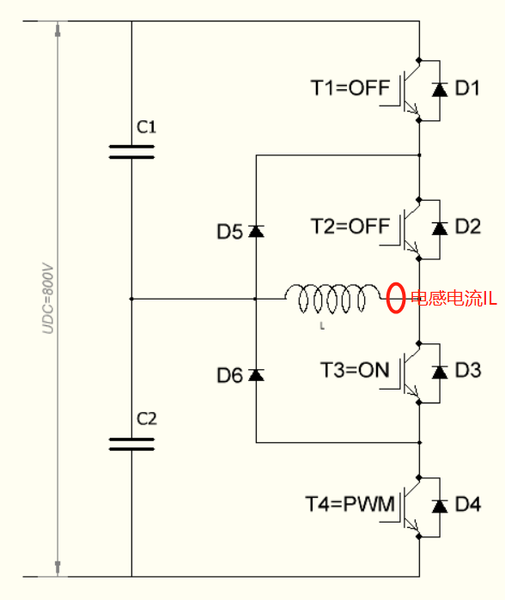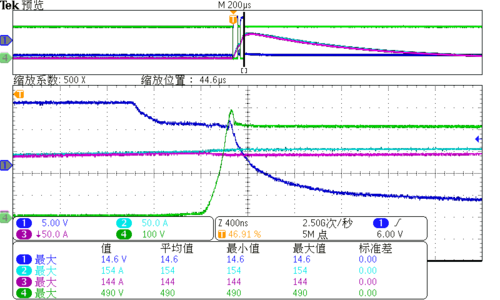（VUDC=800V，IL =150A，CH1=VGE-T4 ，CH2=CH3=IL，CH4=VCE-T4 =490V）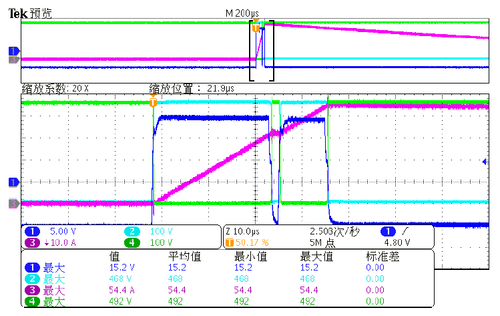（VUDC=900V， CH1=VGE-T4 ，CH2= VCE-D6=468V，CH3=IL，CH4=VCE-T4

2、驱动器高压双脉冲T2管测试波形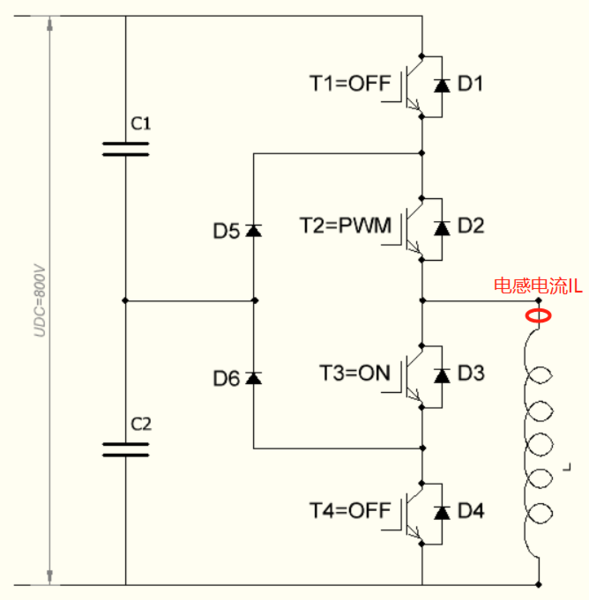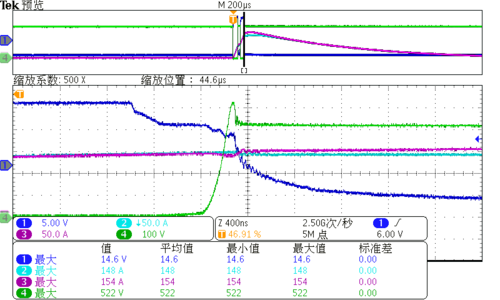（VUDC=800V，IL =150A，CH1=VGE-T2 ，CH2=CH3=IL，CH4=VCE-T2 =522V）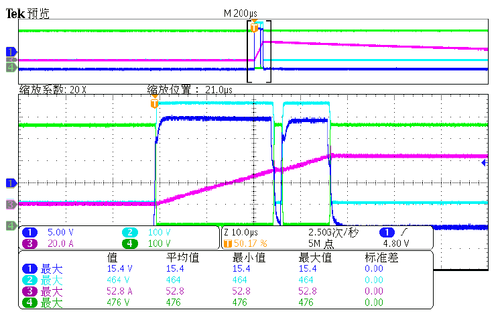（VUDC=900V， CH1=VGE-T2 ，CH2= VCE-D4=464V，CH3=IL，CH4=VCE-T2

3、驱动器高压短路T4管测试波形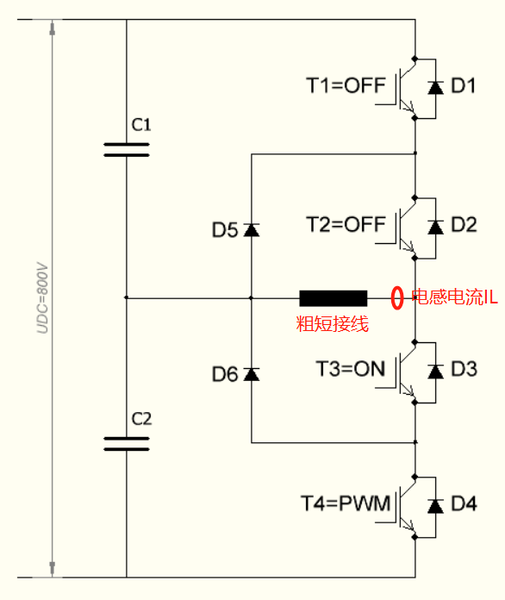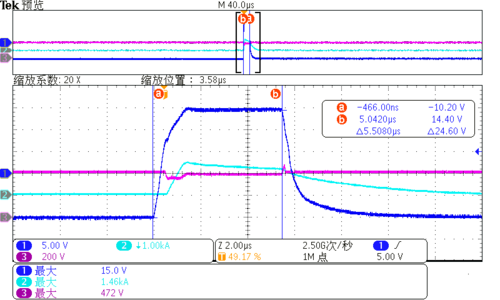（VUDC=800V， CH1=VGE-T4 ，CH2 =2IL=1.46KA，CH3=VCE-T4=472V）

4、驱动器高压短路T2管测试波形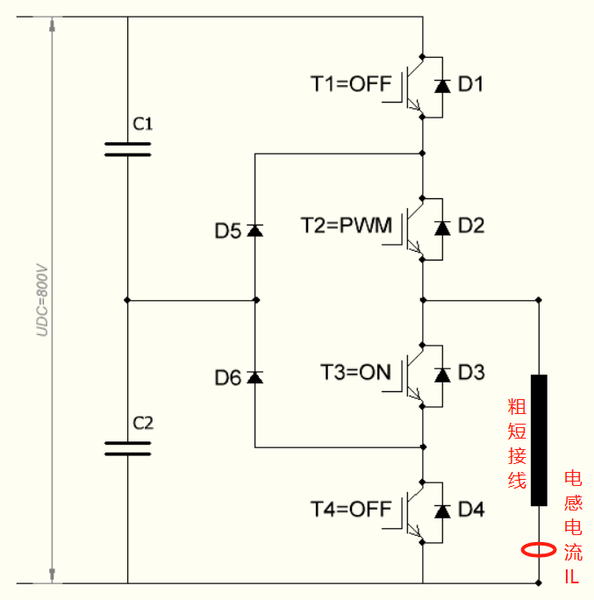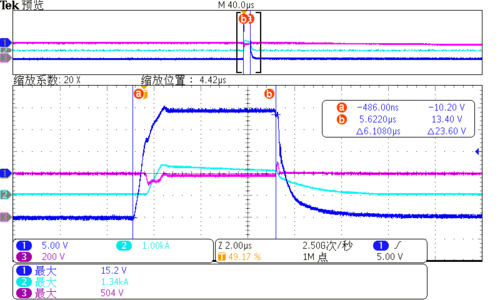（VUDC=800V， CH1=VGE-T2 ，CH2 =2IL=1.34KA，CH3=VCE-T2=504V）